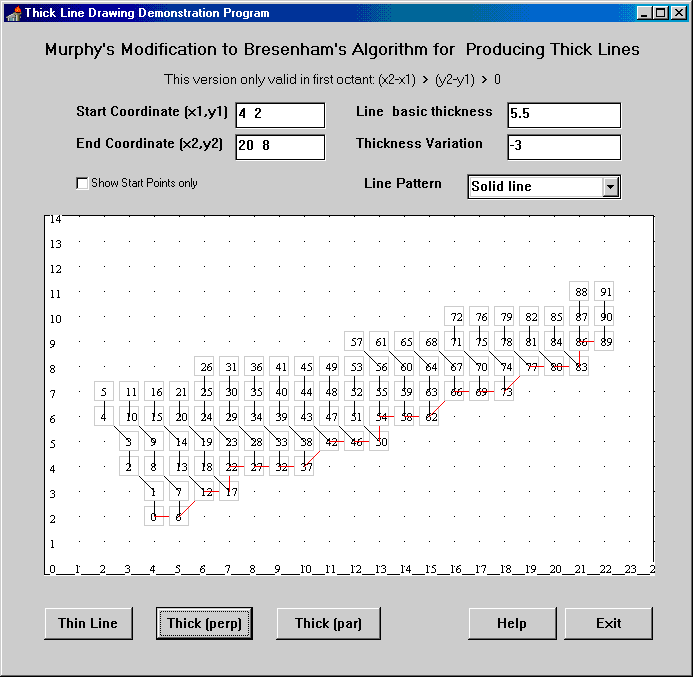## Murphy's Modified Bresenham Line Drawing Algorithm

An Algorithm for drawing thickened lines

### Background

This page describes an algorithm for drawing thickened lines on a display or picture grid. It is based on an extension to Bresenham's Line drawing algorithm - see: "J. E. Bresenham, IBM Systems Journal 4, 25-30 (1965)".

The modified algorithm was first published as 'Line Thickening by Modification to Bresenham's Algorithm' in the IBM Technical Disclosure Bulletin Vol.20 No.12 May 1978 pages 5358-5366. This is copyright IBM Corporation 1978, but IBM have kindly granted their permission for me to reproduce the article here (each page about 20K):

Page 1
Page 2
Page 3
Page 4
Page 5
Page 6
Page 7
Page 8
Page 9

• Each pixel, which is set, is visited only ONCE - this makes the algorithm very suitable for use in XOR situations (for exampe, when pixels are XORed onto other images).
• The inner loop and most of the outer loop require only addition, and comparision testing. This produces an efficient algorithm.
• The line thickness can be a real number, is is not necessary to be an integer.
• The perceived line thickness does not vary for lines of different orientations.
• It is possible to have a thickness which is a function of the distance from the start point - the thickness varies along the line, giving the possibility of depth-cueing (Z-axis effects).

#### General Principle

In order to draw a thick line, you need to draw a number of parallel single thickness lines. There are 2 ways to do this:

1. Draw lines perpendicular to the ideal line stepping along it.
2. Draw lines parallel to the ideal line and step perpendicularly.

The document describes algorithms for each of the above situations. Both algorithms have two Bresehham loops, one inside the other. The outer loop does the 'stepping' and the inner loop draws single pixel lines.

The key aspect of the technique is that the outer loop contains just the right information for the inner loop. This is important as the inner loop must be started in the 'correct' phase of its cycle so that the diagonal moves occur in phase. The outer loop's difference terms are passed to the inner loop to accomplish this.

Another aspect of the technique is that an 'extra' inner loop cycle is required whenever the outer loop and the inner loop do a diagonal move together. In this case the outer loop does a 'double square' move rather than a diagonal move. When the outer loop detects this condition it performs a square move, calls the inner loop, does another square move at right angles and calls the inner loop again. In this way a diagonal move is achieved but there are two calls to the inner loop.

#### Demonstration Program

The original paper is not particularly clear. However, I have produced a thick line drawing demonstration program which better illustrates the technique (115K approx). This is an Object Pascal Delphi 1 program complete with source code.

I have rushed the programming and so the demo is NOT well tested. Please be careful if you run it by saving all important data in other programs first. Because the loop end conditions are tricky to get right, it is just possible that the demo may get into an infinite loop. This version of the demo only supports the drawing of lines in the first octant - where (X2-X1)>(Y2-Y1)>0. Drawing lines in other octants is obtained by defining the 4 types of moves required by the loops. I hope to illustrate this in a future version of the demo. Also the thick line is drawn to one side of the ideal line. This will be corrected in a future version.

#### Variations

The demo program illustrates one method by which line patterns may be formed. In this case the pattern is a function of (p,q) and so the pattern is with respect to the line orientation. An alternative is to make the pattern a function pt.x and pt.y - this produces slightly nicer looking patterns. The line thickness variation is illustrated using the 'thickfunct' which shows how the thickness can be varied along the length of the line. This uses the Thick(Perp) algorithm.

#### Screen Shot

The following is a screen shot of the demonstration program:This shows a line drawn from (4 2) to (20 8) with a line thickness of 5.5 and a reducing taper of -3, using the Thick(perp) algorithm. It shows the sequence order in which the pixels are drawn (0,1,2,3.....).

Note that between line y=5 and y=6 all perpendicular lines execute the diagonal move 'in phase' with each other.

The start points for the perpendicular lines are 0,6,12,17,22,27,32,37,42,46...... Note that the pixel labelled 22 is 'extra' to Bresnemham's original algorithm in that it is produced by a double square move rather than a diagonal move between pixels labelled 17 and 27. This extra line is required because the outer loop executes a diagonal move whilst the inner loop does so as well (between lines y=5 and y=6). This extra pixel produces the perpendicular line (pixels 22-26).

#### Warning

Because the demo program is NOT well tested, the author accepts no responsibility for the operation of the algorithm in its current state.

#### Contacting me